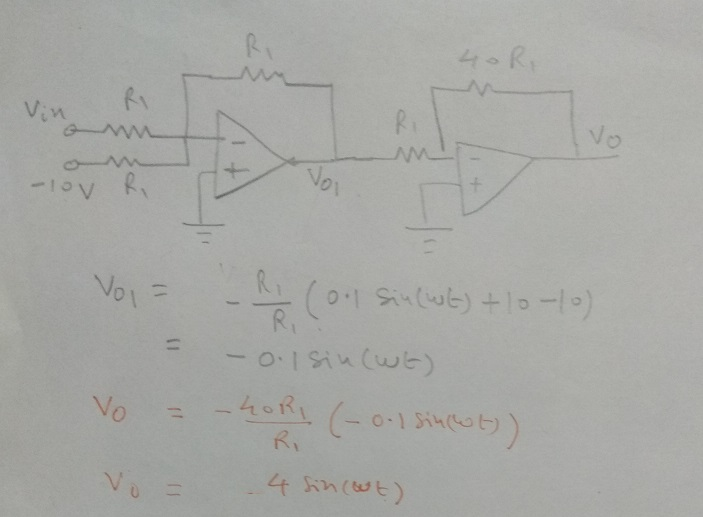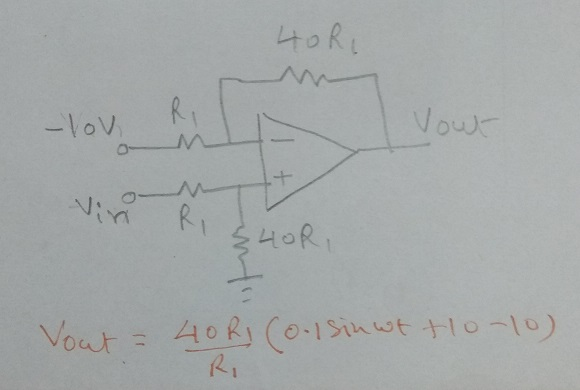Homework Help Question & Answers

1. We wish to sample a small signal that is on top of a large DC offset using a DAQ (Digital Acqu...1. We wish to sample a small signal that is on top of a large DC offset using a DAQ (Digital Acquisition System such as your nscope) that has a limited input voltage range of ±5 V. If the signal is modeled as Vin-0.1sin(ot)+10 V where 0.1sin(ot) V is the signal and 10V is the DC offset, design a circuit using two op-amps that first removes the DC offset then amplifies the signal by a factor of 40. 2. Repeat problem I but using only one op-amp.

In this case use an adder as the first stage to get rid on 10V offset (we add -10V to the input signal).Then we use an inverting amplifier as the second stage to get a gain of 40X. It also removes the negative sign introduced by the adder stage.One can take R1 = 1K, therefore 40*R1 = 40K. One can use 39K + 1K to make 40K resistance if one needs to use commercially available resistance values.

Answer 2) In this case we use a differential amplifier of gain 40. It subtracts -10V as well as amplifies the difference by 40X.Hope this helps.

Add Answer of: 1. We wish to sample a small signal that is on top of a large DC offset using a DAQ (Digital Acqu...
More Homework Help Questions Additional questions in this topic.

• 2. G-proteins. How do G-proteins act as signal transducers and amplify a signal? 2. G-proteins. How do G-proteins act as signal transducers and amplify a signal?

Need Online Homework Help?

Get FREE EXPERT Answers
WITHIN MINUTES
Related Questions